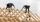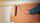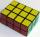# Cuboid - edges

The cuboid has dimensions in ratio 4: 3: 5, the shortest edge is 12 cm long. Find:
(A) the lengths of the remaining edges,
(B) the surface of the cuboid,
(C) the volume of the cuboid

Result

a =  16 cm
c =  20 cm
S =  1504 cm2
V =  3840 cm3

#### Solution:Leave us a comment of example and its solution (i.e. if it is still somewhat unclear...):

Showing 0 comments:Be the first to comment!#### To solve this example are needed these knowledge from mathematics:

Tip: Our volume units converter will help you with converion of volume units.

## Next similar examples:

1. Surface of cuboidFind the surface of the cuboid if its volume is 52.8 cm3 and the length of its two edges is 2 cm and 6 cm.
2. Cuboid - VabFind the surface of the cuboid when its volume is 52.8 cubic centimeters, and the length of its two edges is 2 centimeters and 6 centimeters.
3. Cuboid surfaceDetermine surface area of cuboid if its volume is 52.8 cm cubic and length of the two edges are 2 cm and 6 cm.
4. Water levelThe glass container has a cuboid shape with dimensions of the bottom 24 cm and 12 cm. The height of water is 26 cm. Calculate the volume of the body, which are sunk into the water if the water level rise by 3 cm.
5. CarpenterFrom wooden block carpenter cut off a small cuboid block with half the edge length. How many percent of wood he cut off?
6. Cuboid enlargementBy how many percent increases the volume of cuboid if its every dimension increases by 30%?
7. CuboidCuboid has a surface of 516 cm2. Side a = 6 cm and b = 12 cm. How long is the side c =?
8. Water in aquariumThe aquarium cuboid shape with a length of 25 cm and a width of 30 cm is 9 liters of water. Calculate the areas which are wetted with water.
9. PainterHow many euros we will pay for repainting the room shaped cuboid with a length of 4.5 meters, width of 2.5 meters and a height of 3 meters, if for 1 m2 with paint we pay € 1.5?
10. Cuboid - simpleCalculate the surface area and volume of a cuboid if a = 8 cm, b = 14 cm and c = 6 cm.
11. The volumeThe volume of a solid cylinder is 260 cm3 the cylinder is melt down into a cuboid, whose base is a square of 5cm, calculate the height of the cuboid and the surface area of the cuboid
12. Cube 1-2-3Calculate the volume and surface area of the cube ABCDEFGH if: a) /AB/ = 4 cm b) perimeter of wall ABCD is 22 cm c) the sum of the lengths of all edges of the cube is 30 cm.
13. Cube wallsFind the volume and the surface area of the cube if the area of one of its walls is 40 cm2.
14. Cube volumeThe cube has a surface of 384 cm2. Calculate its volume.
15. Simplify 2Simplify expression: 5ab-7+3ba-9
16. Holidays - on poolChildren's tickets to the swimming pool stands x € for an adult is € 2 more expensive. There was m children in the swimming pool and adults three times less. How many euros make treasurer for pool entry?
17. Garden pondConcrete garden pond has bottom shape of a semicircle with a diameter 1.7 m and is 79 cm deep. Daddy wants make it surface. How many liters of water is in pond if watel level is 28 cm?